A bit on missing data:

When we have missing values in a dataset it is important to think about why they are missing and their impact on analysis. Sometimes ignoring missing data reduces power, but more importantly, sometimes it biases answers and potentially misleads to incorrect conclusions. So it is important to think about what the missing data mechanism is in order to see what to do about it. Rubin (1976) differentiated between three types of missigness mechanisms:

1. Missing completely at random (MCAR): when cases with missing values can be thought of as a random sample of all the cases; MCAR occurs rarely in practice.

2. Missing at random (MAR): when conditioned on all the data we have, any remaining missingness is completely random; that is, it does not depend on some missing variables. So missingness can be modelled using the observed data. Then, we can use specialised missing data analysis methods on the available data to correct for the effects of missingness.

3. Missing not at random (MNAR): when data is neither MCAR nor MAR. This is difficult to handle because it will require strong assumptions about the patterns of missingness.

One common way people try to deal with missing data is to delete all cases for which a value is missing. This method is called complete case analysis (CC). However, CC is valid only if data is MCAR. Another method is multiple imputation (MI), which is a monte carlo method that simulates multiple values to impute (fill-in) each missing value, then analyses each imputed dataset separately and finally pools the results together. We impute missing data multiple times to account for uncertainty we have about the true (& unknown) values of the missing data. We will get more comfortable with MI as we work with the example dataset. In theory, MI can handle all the three types of missingness. However, packages that do MI are usually not designed for MNAR case. Missing data analysis of MNAR data is more complicated and is not the focus of this lab. Here we work with a dataset where we are assuming the data are MAR.

# required libraries
library(mice)
## Warning: package 'mice' was built under R version 3.1.3
## Loading required package: Rcpp
## Warning: package 'Rcpp' was built under R version 3.1.3
## Loading required package: lattice
## mice 2.22 2014-06-10
library(VIM)
## Warning: package 'VIM' was built under R version 3.1.3
## Loading required package: colorspace
## Warning: package 'colorspace' was built under R version 3.1.3
## Loading required package: grid
## Loading required package: data.table
## Warning: package 'data.table' was built under R version 3.1.3
## VIM is ready to use.
##  Since version 4.0.0 the GUI is in its own package VIMGUI.
##
##           Please use the package to use the new (and old) GUI.
##
## Suggestions and bug-reports can be submitted at: https://github.com/alexkowa/VIM/issues
##
## Attaching package: 'VIM'
##
## The following object is masked from 'package:datasets':
##
##     sleep
library(lattice)

Dataset: Example data is simulated based on the Phase 1 of the Third National Health and Nutrition Examination Survey (NHANES) by the US National Center for Health Statistics. The study was designed to assess the health and nutritional status of the population by subgroups such as of age. Age was obtained through a household screening interview from the individuals themselves or neighbours if they were not present. Thus, age is fully observed. Health stata were collected through actual physical examinations of the sampled persons in mobile examination centers. Some of the individuals did not show up and their absence is assumed to be mostly due to inability to participate in certain examination procedures, and thus missingness is assumed to only depend on fully observed variable age (a MAR case). Missing proportions are between 30% to 40%.

# load data
data(nhanes)
str(nhanes)
## 'data.frame':    25 obs. of  4 variables:
##  $age: num 1 2 1 3 1 3 1 1 2 2 ... ##$ bmi: num  NA 22.7 NA NA 20.4 NA 22.5 30.1 22 NA ...
##  $hyp: num NA 1 1 NA 1 NA 1 1 1 NA ... ##$ chl: num  NA 187 187 NA 113 184 118 187 238 NA ...
# contains 25 obs & four variables: age (age groups: 20-39, 40-59, 60+), bmi (body mass index),
# hyp (hypertension status) and chl (cholesterol level).
nhanes$age = factor(nhanes$age)

Visual inspection of the missing data:

# We can see the pattern of the missing data in mice package by
md.pattern(nhanes) 
##    age hyp bmi chl
## 13   1   1   1   1  0
##  1   1   1   0   1  1
##  3   1   1   1   0  1
##  1   1   0   0   1  2
##  7   1   0   0   0  3
##      0   8   9  10 27
# 5 patterns observed from 2^3 possible patterns; we see for example that there are 3 cases where chl is missing whereas all the other variables are observed.

# Missingness pattern can also be visualised in VIM package by
library(VIM)
nhanes_aggr = aggr(nhanes, col=mdc(1:2), numbers=TRUE, sortVars=TRUE, labels=names(nhanes), cex.axis=.7, gap=3, ylab=c("Proportion of missingness","Missingness Pattern"))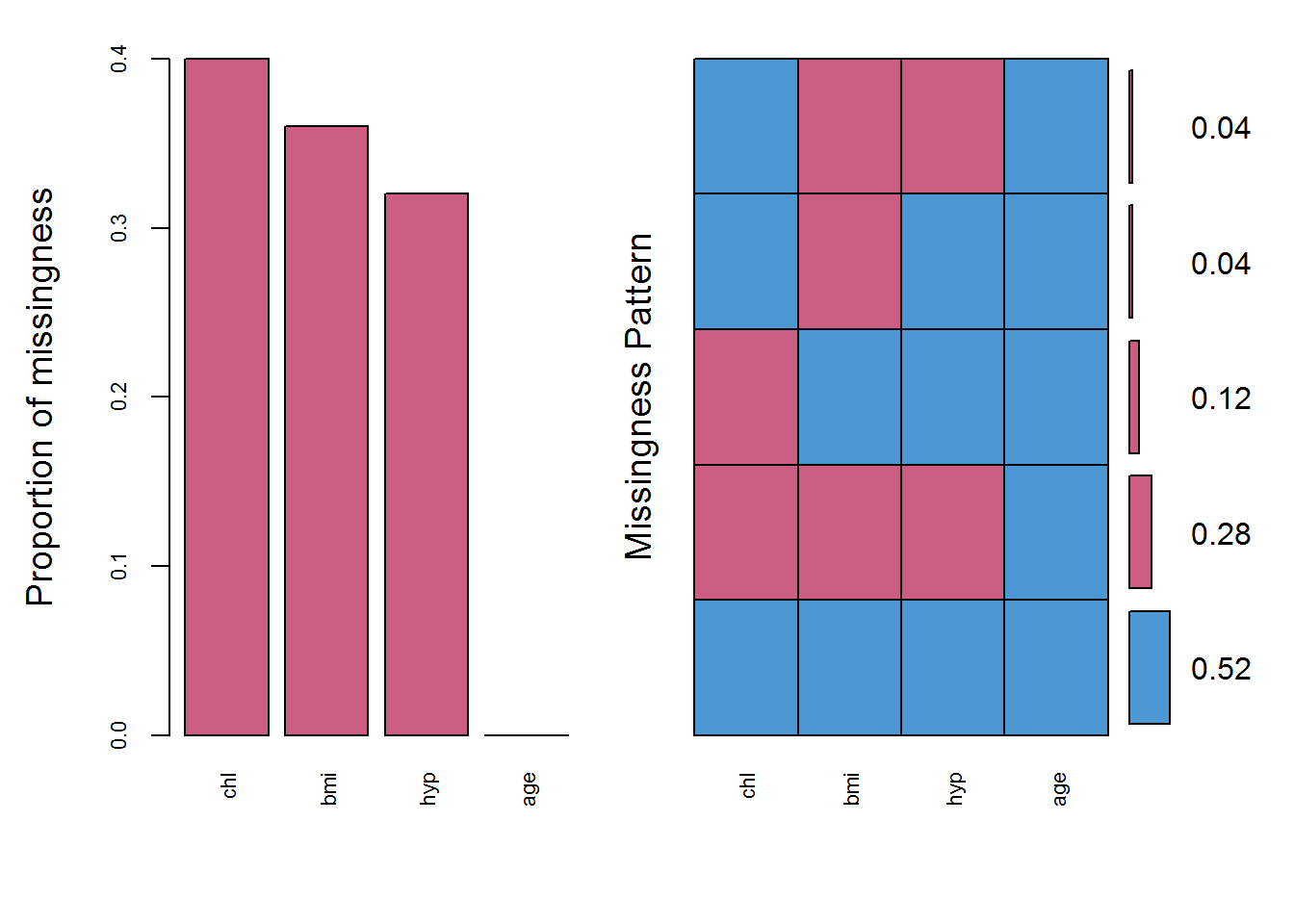##
##  Variables sorted by number of missings:
##  Variable Count
##       chl  0.40
##       bmi  0.36
##       hyp  0.32
##       age  0.00
# This plot gives the frequencies for different combination of variables missing. For example,
# that all the three variables chl, bmi & hyp are missing is the most frequent with about 28% frequency (7 observations). Note that, blue refers to observed data and red to the missing data.

# You can also try it in mi package by
# missing.pattern.plot(nhanes, mis.col=mdc(2), obs.col=mdc(1), main="Misisng Pattern")
# The margin plot of the pairs can be plotted using VIM package as
marginplot(nhanes[, c("chl", "bmi")], col = mdc(1:2), cex.numbers = 1.2, pch = 19)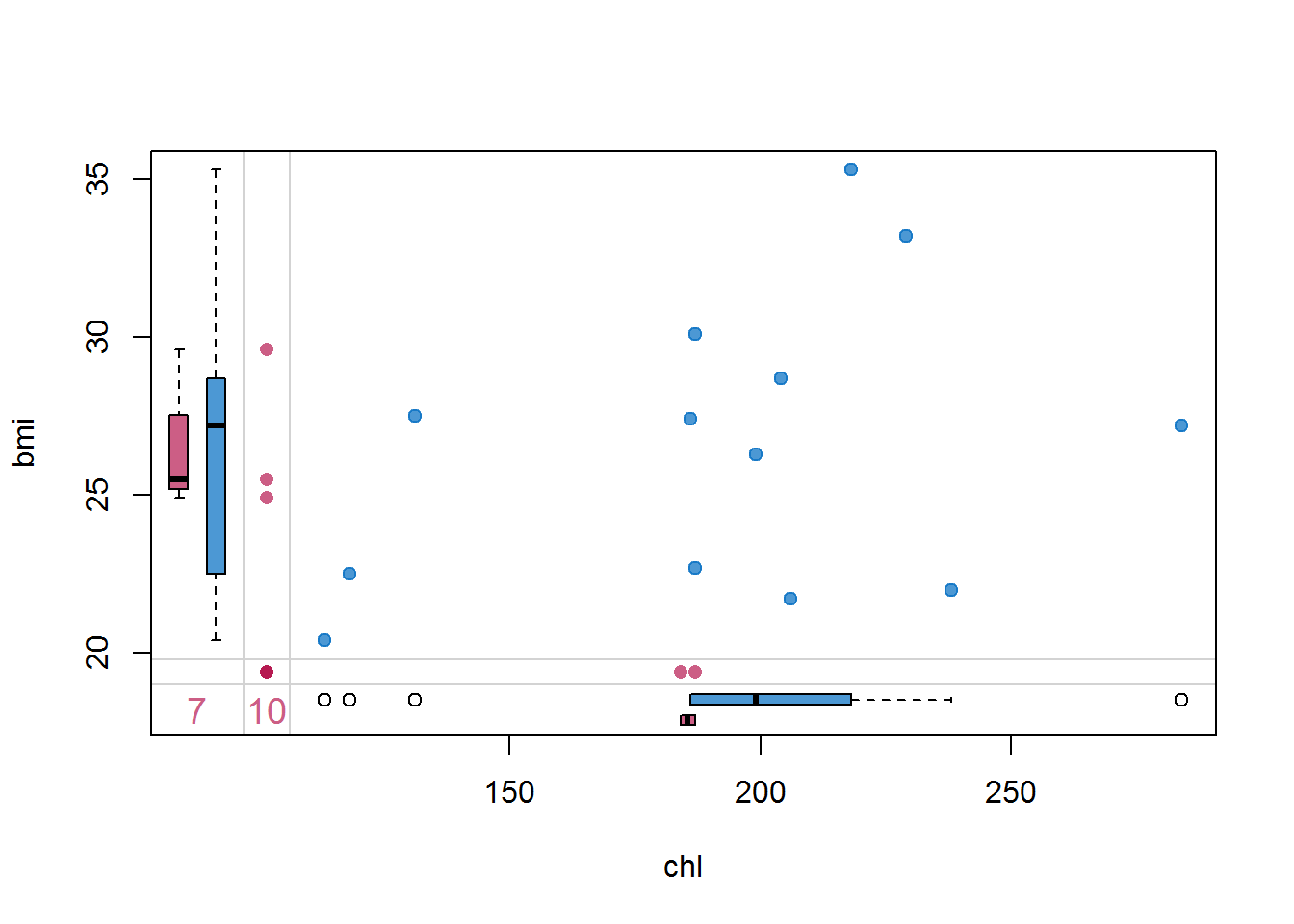# blue box plots summarise the distribution of observed data given the other variable is observed,
# and red box plots summarise the distribution of observed data given the other variable is missing.
# For example, the red box plot in the bottom margin shows that bmi is rather missing for lower cholesterol levels.
# Note that, if data are MCAR, we expect the blue and red box plots to be identical.

## If interested, you can also use VIM for scatterplots as
# scattMiss(nhanes[,c("bmi","chl")], inEllipse=TRUE, col=mdc(1:2),alpha=.8,bty="n", interactive=TRUE, axes=TRUE, lwd=c(1.5,1.5), pch=19, cex.lab=1.1, cex.axis=.9)
# rugNA(nhanes[,c("bmi")], nhanes[,c("chl")], side=2, col=mdc(2), lwd=1.5)
# rugNA(nhanes[,c("bmi")], nhanes[,c("chl")], side=1, col=mdc(2), lwd=1.5)

How to handle the missing data?

# We are interested in the simple linear regression of chl on age and bmi

# The naive approach:
# Delete cases with missing values (complete case analysis), and fit a linear model,
# lm function does these two steps together, however, remember that it is not a valid method for MAR data.
fit.cc = lm(chl ~ age + bmi, data=nhanes)
summary(fit.cc)
##
## Call:
## lm(formula = chl ~ age + bmi, data = nhanes)
##
## Residuals:
##     Min      1Q  Median      3Q     Max
## -30.504 -19.967   0.755   7.619  58.871
##
## Coefficients:
##             Estimate Std. Error t value Pr(>|t|)
## (Intercept)  -28.948     56.221  -0.515  0.61903
## age2          55.810     18.418   3.030  0.01424 *
## age3         104.724     24.843   4.215  0.00225 **
## bmi            6.921      1.951   3.548  0.00624 **
## ---
## Signif. codes:  0 '***' 0.001 '**' 0.01 '*' 0.05 '.' 0.1 ' ' 1
##
## Residual standard error: 29.1 on 9 degrees of freedom
##   (12 observations deleted due to missingness)
## Multiple R-squared:  0.7329, Adjusted R-squared:  0.6439
## F-statistic: 8.232 on 3 and 9 DF,  p-value: 0.006013
# bmi and age both significant, however, based only on 13 observations (12 observations are deleted).

## Fill-in (impute) missing values by MI method

# One way to do that is using mice package
library(mice)

What does mice do?

                                mice() ----> with() ----> pool()
• mice() imputes each missing value with a plausible value (simulates a value to fill-in the missing one) until all missing values are imputed and dataset is completed. Repeats the process for multiple times, say m times and stores all the m complete(d)/imputed datasets.

• with() analyses each of the m completed datasets separately based on the analysis model you want.

• pool() combines (pools) all the results together based on Rubin’s rules (Rubin, 1987).

Run MI by mice:

# Function mice() in mice package is a Markov Chain Monte Carlo (MCMC) method that uses
# correlation structure of the data and imputes missing values for each incomplete
# variable m times by regression of incomplete variables on the other variables iteratively.
imp = mice(nhanes, m=5, printFlag=FALSE, maxit = 40, seed=2525)
# The output imp contains m=5 completed datasets. Each dataset can be analysed
# using function with(), and including an expression for the statistical analysis approach
# we want to apply for each imputed dataset as follows
fit.mi = with(data=imp, exp = lm(chl ~ age + bmi))

# Next, we combine all the results of the 5 imputed datasets using the pool() function
combFit = pool(fit.mi)
# Note that the function pool() works for any object having BOTH coef() and vcov() methods, such as lm, glm and Arima, also for lme in nlme package.
round(summary(combFit),2)
##               est    se    t    df Pr(>|t|)   lo 95  hi 95 nmis  fmi
## (Intercept)  6.39 68.44 0.09  7.17     0.93 -154.68 167.46   NA 0.55
## age2        44.86 17.29 2.59 13.33     0.02    7.60  82.13   NA 0.30
## age3        59.51 22.16 2.69  6.84     0.03    6.86 112.16   NA 0.56
## bmi          5.93  2.37 2.51  7.40     0.04    0.40  11.47    9 0.54
##             lambda
## (Intercept)   0.44
## age2          0.20
## age3          0.45
## bmi           0.42
# This result shows that bmi and age are significant. But is m=5 imputed datasets sufficient? Maybe, but if possible, it would be better to increase it to check, e.g. m=20:
# Increase the number of imputations to m=20
imp20 = mice(nhanes, m=20, printFlag=FALSE, maxit = 30, seed=2525)
fit.mi20 = with(data=imp20, exp = lm(chl ~ age + bmi))
combFit = pool(fit.mi20)
round(summary(combFit),2)
##               est    se    t    df Pr(>|t|)   lo 95  hi 95 nmis  fmi
## (Intercept)  4.99 58.22 0.09 15.39     0.93 -118.84 128.81   NA 0.27
## age2        45.99 19.14 2.40 12.69     0.03    4.53  87.44   NA 0.39
## age3        68.41 23.40 2.92  9.53     0.02   15.92 120.91   NA 0.54
## bmi          5.93  1.98 2.99 16.22     0.01    1.74  10.12    9 0.23
##             lambda
## (Intercept)   0.18
## age2          0.30
## age3          0.45
## bmi           0.14
# The results are not much changed. MI works for as low as m=5 for this example.

# You can also see the pooled adjusted R-squared as
pool.r.squared(fit.mi)
##           est     lo 95     hi 95       fmi
## R^2 0.4803841 0.1091925 0.7698717 0.3639035

Are imputations created by mice plausible?

# Check for implausible imputations (values that are clearly impossible, e.g. negative values for bmi)
# The imputations, for example for bmi, are stored as
imp$imp$bmi
##       1    2    3    4    5
## 1  29.6 28.7 20.4 27.2 28.7
## 3  29.6 28.7 30.1 30.1 28.7
## 4  27.5 22.7 25.5 27.5 27.4
## 6  27.4 22.7 22.7 27.4 22.7
## 10 22.7 27.5 22.0 22.5 27.5
## 11 25.5 29.6 27.2 28.7 28.7
## 12 25.5 28.7 22.5 27.4 22.0
## 16 27.5 28.7 28.7 35.3 30.1
## 21 20.4 22.7 22.5 26.3 30.1
# Completed datasets (observed and imputed), for example the second one, can be extracted by
imp_2 = complete(imp, 2)

Checking imputations visually:

# We can inspect the distributions of the original and the imputed data:

## scatterplot (of chl and bmi) for each imputed dataset
xyplot(imp, bmi ~ chl | .imp, pch = 20, cex = 1.4)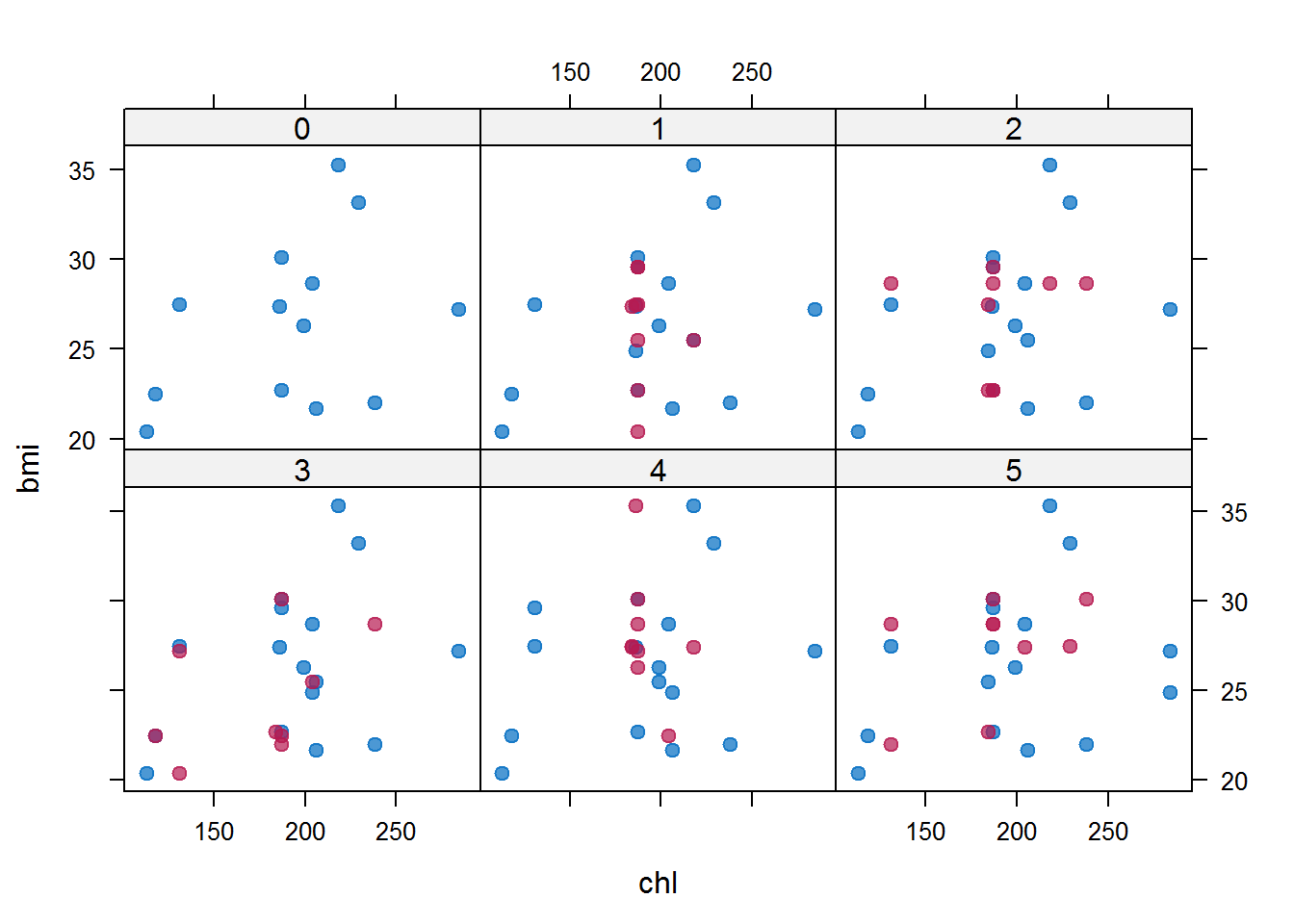# Blue represents the observed data and red shows the imputed data. These colours are consistent with what they represent from now on.
# Here, we expect the red points (imputed data) have almost the same shape as blue points
# (observed data). Blue points are constant across imputed datasets, but red points differ
# from each other, which represents our uncertainty about the true values of missing data.

## To detect interesting differences between observed and imputed data
densityplot(imp)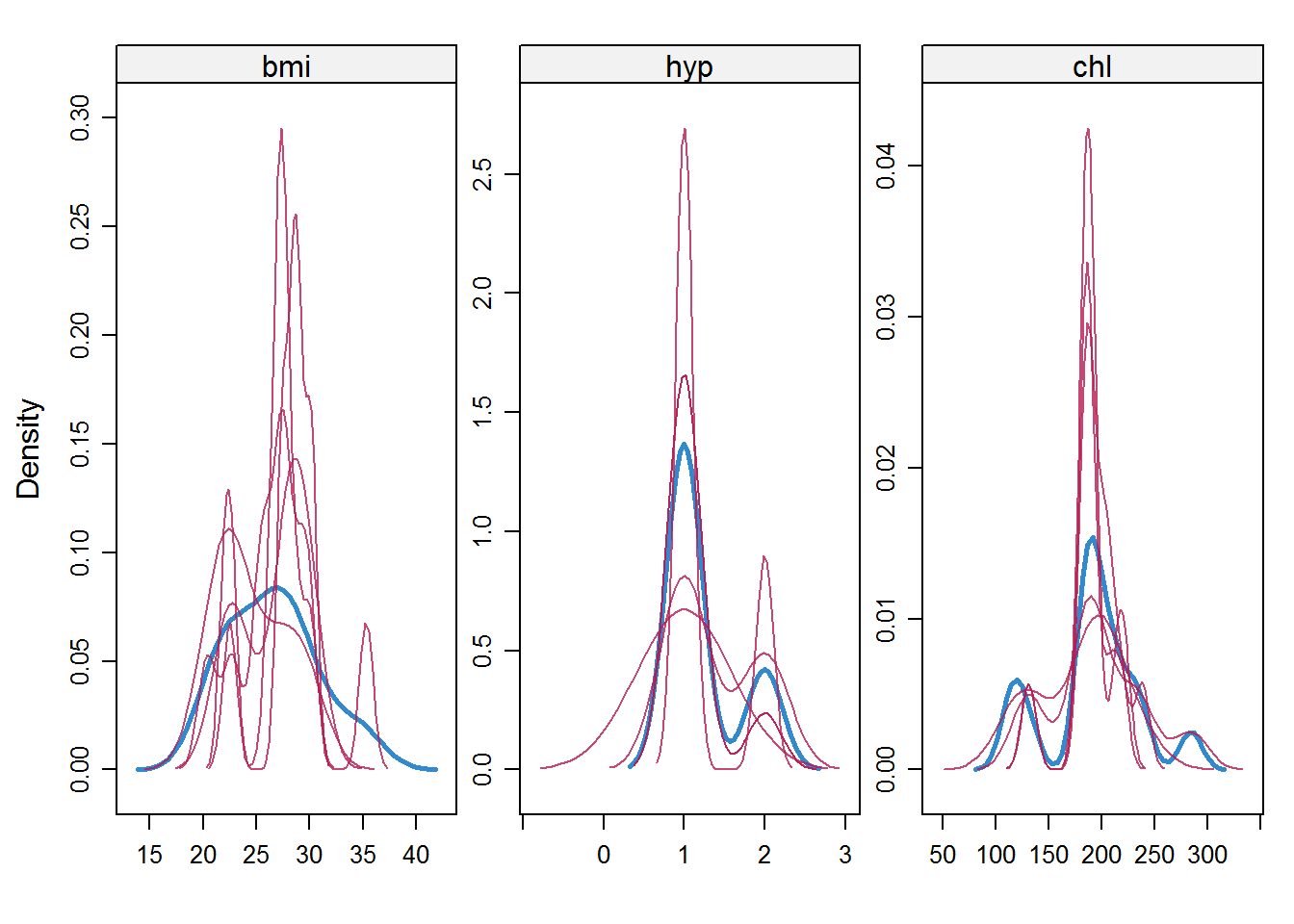# This plot compares the density of observed data with the ones of imputed data. We expect them
# to be similar (though not identical) under MAR assumption.

# Distributions of the variables as individual points can be visualised by
# stripplot(imp, pch = 20, cex = 1.2); imputations should be close to the data; we do not expect to see see impossible values like for example negative values for bmi or 1.5 for hyp

Convergence monitoring:

# MICE runs m parallel chains, each with a certain number of iterations, and imputes
# values from the final iteration. How many iterations does mice() use and how can we make sure that
# this number is enough?
# To monitor convergence we can use trace plots, which plot estimates against the number of iteration.
plot(imp20)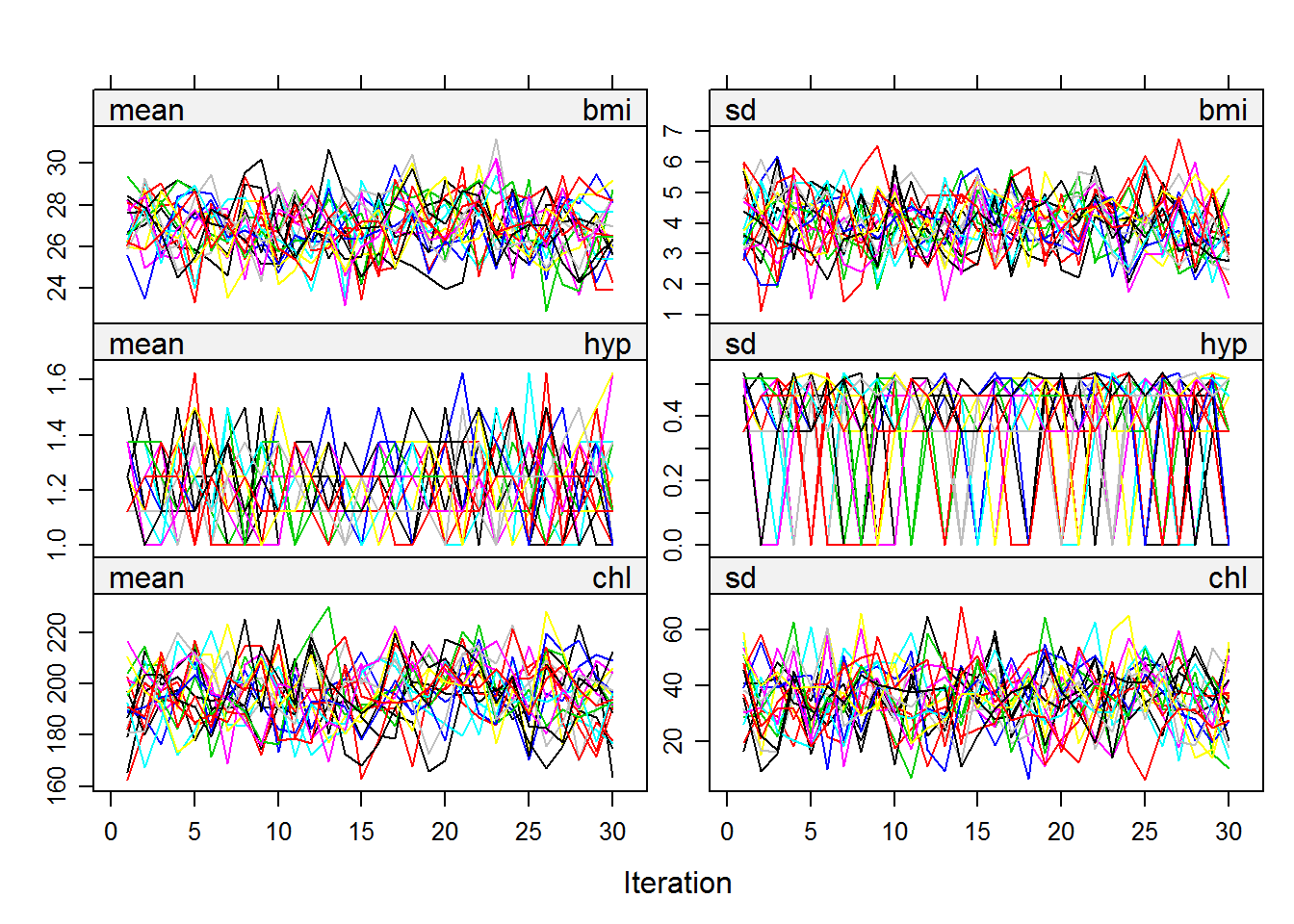# shows mean and standard deviation of the variables through the iterations for the m
# imputed datasets. An indicator of convergence is how well the m parallel chains mix. We can also see here for example that mice has treated hyp as a continuous variable.

How did mice() actually impute values?

# See the univariate imputation model for each incomplete variable that mice() used
# for your data as default
imp$meth ## age bmi hyp chl ## "" "pmm" "pmm" "pmm" pmm stands for predictive mean matching, default method of mice() for imputation of continous incomplete variables; for each missing value, pmm finds a set of observed values with the closest predicted mean as the missing one and imputes the missing values by a random draw from that set. Therefore, pmm is restricted to the observed values, and might do fine even for categorical data (though not recommended). # Possible imputation models provided by mice() are methods(mice) ## Warning in methods(mice): function 'mice' appears not to be generic ##  mice.impute.2l.norm mice.impute.2l.pan ##  mice.impute.2lonly.mean mice.impute.2lonly.norm ##  mice.impute.2lonly.pmm mice.impute.cart ##  mice.impute.fastpmm mice.impute.lda ##  mice.impute.logreg mice.impute.logreg.boot ##  mice.impute.mean mice.impute.norm ##  mice.impute.norm.boot mice.impute.norm.nob ##  mice.impute.norm.predict mice.impute.passive ##  mice.impute.pmm mice.impute.polr ##  mice.impute.polyreg mice.impute.quadratic ##  mice.impute.rf mice.impute.ri ##  mice.impute.sample mice.mids ##  mice.theme # For example, logreg stands for Bayesian logistic regression for binary incomplete variable. # You can run ?mice.impute.logreg to get more information whether the imputation model is # suitable for the incomplete variable you want to impute. # Since hyp is binary we want to change the default; also we might want to change the imputation # model for bmi to (Bayesian) normal linear model: meth=imp$meth;
meth = c("", "norm", "logreg", "pmm")
# you might get an error when running mice if the method you specify is not consistent with the
# type of the variable specified in the dataset. So, for this specified method, you also need to
# change the type of hyp to a 2-level factor or a yes/no binary variable.
nhanes$hyp = factor(nhanes$hyp)

Predictor matrix: Which variables does mice() use as predictors for imputation of each incomplete variable?

imp$pred ## age bmi hyp chl ## age 0 0 0 0 ## bmi 1 0 1 1 ## hyp 1 1 0 1 ## chl 1 1 1 0 # by default mice() includes all the variables in the data; it is suggested that we use as many # variables as possible. Do not exclude a variable as predictor from the imputation model if # that variable is going be included in your final analysis model (either as response or predictor) in function with(). # We can specify relevant predictors as follows: # Suppose that hyp is considered irrelevant as a predictor for bmi and chl! pred=imp$pred;
pred[, "hyp"] = 0
pred
##     age bmi hyp chl
## age   0   0   0   0
## bmi   1   0   0   1
## hyp   1   1   0   1
## chl   1   1   0   0

Visiting sequence: In what sequence does mice() impute incomplete variables?

vis=imp$vis; vis ## bmi hyp chl ## 2 3 4 # mice() imputes values according to the column sequence of variables in the dataset, from left # to right. Here, for a specifed iteration of the mice sampler, first bmi is imputed, then # hyp is imputed using the currently imputed bmi and previously imputed chl from the previous # iteration, and so on. # You can choose visiting sequence by increasing order of missing data manually as vis = c(3,2,4) # , or alternatively vis="monotone" # However, if there is no specific pattern in the missingness (we say the missingness pattern # is general of arbitrary), we do not expect the visiting sequence to affect the results much. Model testing: # mice provides Wald test for testing models. It is also possible to do likelihood ratio test if # the final analysis model is logistic regression. You can choose the test in pool.compare() function by "method" option. fit1 = with(data = imp20, expr = lm(chl ~ age)) fit2 = with(data = imp20, expr = lm(chl ~ age + bmi)) # Wald test stat = pool.compare(fit2, fit1, method = "Wald") # P-value of the test stat$p
##             [,1]
## [1,] 0.002859227

Bibliography:

Rubin, D.B., Inference and missing data. Biometrika, 1976. 63(3): p. 581-592.

Rubin, D.B., Multiple Imputation for Nonresponse in Surveys. 1987, New York: John Wiley.

Templ, M., et al., VIM: visualization and imputation of missing values. R package version 2.3, 2011.

Van Buuren, S., Flexible Imputation of Missing Data. 2012, Chapman & Hall/CRC.

Van Buuren, S. and K. Groothuis-Oudshoorn, mice: Multivariate Imputation by Chained Equations in R. Journal of Statistical Software, 2011. 45(3): p. 67.